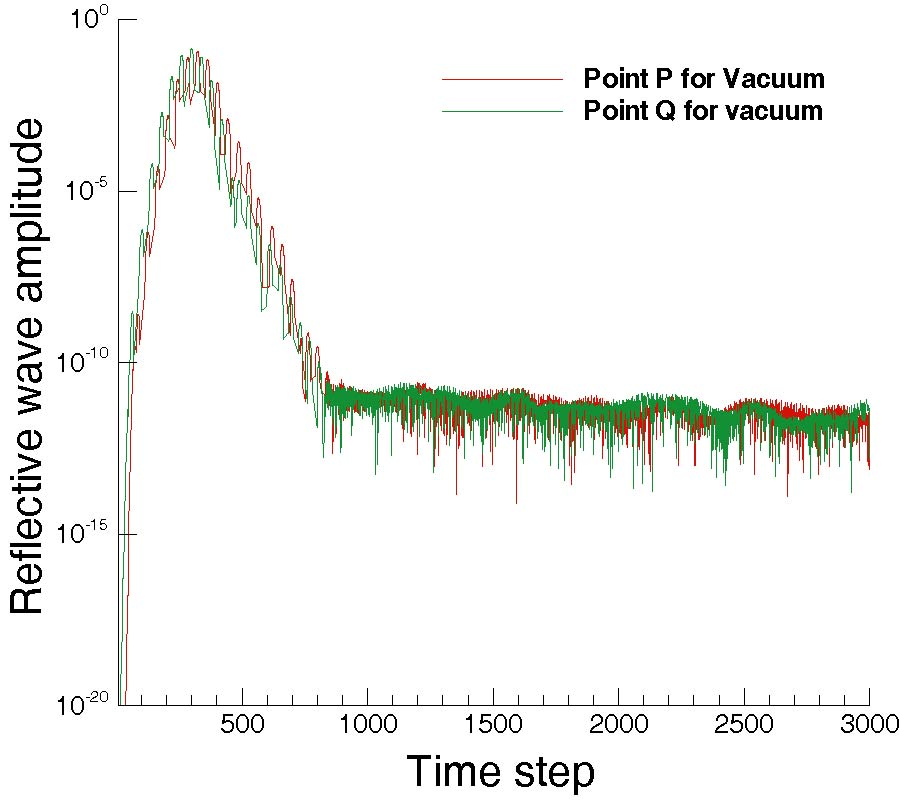# Vol. 132

Front:[PDF file] Back:[PDF file]
Latest Volume
All Volumes
All Issues
2012-10-15

#### Development of a Symplectic Scheme with Optimized Numerical Dispersion-Relation Equation to Solve Maxwell's Equations in Dispersive Media

By Tony Wen-Hann Sheu, Rih Yang Chung, and Jia-Han Li
Progress In Electromagnetics Research, Vol. 132, 517-549, 2012
doi:10.2528/PIER12080901

## Abstract

In this paper an explicit finite-difference scheme is developed in staggered grids for solving the Maxwell's equations in time domain. We are aimed to preserve the discrete zero-divergence condition in the electrical and magnetic fields and conserve the inherent laws in non-dispersive simple media all the time using the explicit second-order accurate symplectic partitioned Runge-Kutta temporal scheme for the time derivative terms. The spatial derivative terms in the semi-discretized Faraday's and Ampere's equations are then approximated to get an accurate numerical dispersion relation equation that governs the numerical angular frequency and the wavenumbers for the Maxwell's equations defined in two space dimensions. To achieve the goal of getting the best dispersive characteristics in the chosen grid stencil, a fourth-order accurate space centered scheme with the ability of minimizing the difference between the exact and numerical dispersion relation equations is proposed. Our emphasis is placed on the accurate modeling of EM waves in the dispersive media of the Debye, Lorentz and Drude types. Through the computational exercises, the proposed dual-preserving Maxwell's equation solver is computationally demonstrated to be efficient for use to predict the long-term accurate wave solutions in a medium belonging either to a frequency independent or to a dependent type.

## Citation

Tony Wen-Hann Sheu, Rih Yang Chung, and Jia-Han Li, "Development of a Symplectic Scheme with Optimized Numerical Dispersion-Relation Equation to Solve Maxwell's Equations in Dispersive Media," Progress In Electromagnetics Research, Vol. 132, 517-549, 2012.
doi:10.2528/PIER12080901
http://jpier.org/PIER/pier.php?paper=12080901

## References

1. Nicolaides, R. A. and D. Q. Wang, "Helicity and variational principles for Maxwell's equations," Int. J. Electron., Vol. 54, 861-864, 1983.
doi:10.1080/00207218308938781

2. Cockburn, B., F. Li, and C. W. Chi, "Locally divergence-free discontinuous Galerkin methods for the Maxwell equations," Journal of Computational Physics, Vol. 194, No. 2, 588-610, 2004.
doi:10.1016/j.jcp.2003.09.007

3. Sheu, W. H., Y. W. Hung, M. H. Tsai, P. H. Chiu, and J. H. Li, "On the development of a triple-preserving Maxwell's equations solver in non-staggered grids ," Int. J. Numer. Meth. Fluids, Vol. 63, 1328-1346, 2010.

4. Sheu, W. H., L. Y. Liang, and J. H. Li, "Development of an explicit symplectic scheme that optimizes the dispersion-relation equation of the Maxwell's equations ," Communications in Computational Physics, Vol. 13, No. 4, 1107-1133, 2013.

5. Yee, K. S., "Numerical solution of initial boundary value problem involving Maxwell's equations in isotropic meida," IEEE Transactions on Antenna Propagation, Vol. 4, No. 3, 302-307, 1966.

6. Roden, J. A. and S. D. Gedney, "Convolutional PML (CPML): An efficient FDTD implementation of the CFS-PML for arbitrary media," Microwave Optical Tech. Lett., Vol. 27, 334-339, 2000.
doi:10.1002/1098-2760(20001205)27:5<334::AID-MOP14>3.0.CO;2-A

7. Berenger, J. P., "A perfectly matched layer for the absorption of electromagnetic waves," Journal of Computational Physics, Vol. 114, No. 2, 185-200, 1994.
doi:10.1006/jcph.1994.1159

8. Taflove, A. and S. C. Hagness, Computational Electrodynamics: The Finite-difference Time-domain Method, 3rd Ed., Artech House, Norwood, MA, 2005.

9. Wei, B., S. Q. Zhang, F. Wang, and D. Ge, "A novel UPML FDTD absorbing boundary condition for dispersive media, waves in random and complex media," Journal of Mathematical Physics, Vol. 20, No. 3, 511-527, 2010.

10. Luebbers, R. J., F. P. Huusberger, K. S. Kunz, R. B. Standler, and M. Schneider, "A frequency-dependent finite-difference time-domain formulation for dispersive materials," IEEE Transactions on Electromagnetic Compatibility, Vol. 32, No. 3, 222-227, 1990.
doi:10.1109/15.57116

11. Anderson, N. and A. M. Arthurs, "Helicity and variational principles for Maxwell's equations," Int. J. Electron, Vol. 54, 861-864, 1983.
doi:10.1080/00207218308938781

12. Gao, L., B. Zhang, and D. Liang, "The splitting finite-difference time-domain methods for Maxwell's equations in two dimensions," J. Comput. Applied Math, Vol. 205, 207-230, 2007.
doi:10.1016/j.cam.2006.04.051

13. Wei, B., X. Y. Li, F. Wang, and D. Ge, "A finite difference time domain absorbing boundary condition for general frequency-dispersive media," Acta Physica Sinica, Vol. 58, No. 7, 6174-6178, 2009.

14. Cole, K. S. and R. H. Cole, "Dispersion and absorption in dielectrics," J. Chem. Phys, Vol. 9, 341, 1941.
doi:10.1063/1.1750906

15. Kelley, F. and R. J. Luebber, "Piecewise linear recursive convolution for dispersive media using FDTD," IEEE Transactions on Electromagnetic Compatibility, Vol. 44, No. 6, 1966.